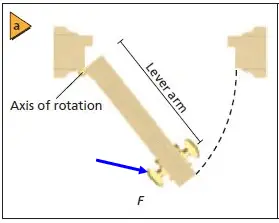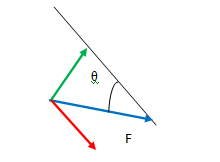# PhysicsTeacher.in

## High School Physics BLOG - online class notesRSS Feed

## Torque definition physics

Torque formula and Torque meaning in physics: Torque is the rotational analogue of force.

In other words, as force causes translational motion, the Torque causes rotational motion.

We can’t separate a torque from a force as it is not possible to apply a torque without applying a force.

Torque is the measure of how effectively a force can twist or turn something. That’s why torque is also known as Moment of Force.(Rotational domain equivalent of Force).

If we take the example of bicycle wheel that rotates about a fixed axis, then we can say that a torque can change the rotational motion either by making it rotate faster or by making the rotation slower.

If the net torque is zero then a body will not change its rotational state of motion. Torque is denoted as T or τ (Tao).

In this article we will cover the Torque Formula with practical examples and diagrams.
*We will come up with the Torque Formula with the understanding that it is the moment of force.
*Also we will show you how to derive formula of torque, with the understanding that torque is the rate of change of angular momentum and
*Last one formula for torque will show torque as the cross product of Moment of Inertia and Angular Acceleration

## Torque Formula

1)
Torque formula can be framed considering Torque as the Moment of Force. It is the cross product of Lever Arm Length (r) and the Force applied (F).

T = r x F = r F Sin θ  ………….(1)

Lever arm length r is the shortest distance between the rotation axis and the point of application of the force.

and

θ = angle made between Lever arm and the line of action of the force being applied.

and

F Sin θ is the perpendicular component of the force which is the effective component of the applied force responsible for the rotation.

2) Formula of Torque can also be obtained as the time rate of change of angular momentum.

T = ΔL/ΔT …………(2)

See the derivation below in this post.

3) Formula for torque is available as the cross product of Moment of Inertia(I) and Angular Acceleration(α)

T= I X α   ……………(3)

See the derivation below in this post.

The SI unit for torque is the newton metre (N⋅m).

## Torque meaning in physics and torque formula

We will discuss this with some practical examples. Let’s first consider the Bank Vault door.

Now, What determines a torque produced by a particular force?

When we try to push open a massive bank vault door,certainly we would push as hard as we can.
The torque certainly is proportional to the magnitude of the force.

But just by applying a huge force we can’t expect to get a huge torque.

It also matters where and in what direction we are applying the force.

### In what direction to apply force to get max torque?

For maximum effectiveness, we have to push perpendicularly to the door ( Fig. a above).

If we push radially, i.e. straight in toward the axis of rotation that passes through the hinges, the door wouldn’t rotate, no matter how hard we push ( Fig. b ).

A force acting in any other direction can be decomposed into horizontal and perpendicular components, with the horizontal component contributing nothing to the torque ( Fig. c ). Only the perpendicular component of the force ( F ⊥ ) produces a torque .

### Where to apply the force for maximum torque?

Furthermore, where we apply the force is critical.

Instinctively, we would push at the outer edge, as far from the rotation axis as possible.
If we push close to the axis, it would be difficult to open the door.

Torque is proportional to the distance between the rotation axis and the point of application of the force (the point at which the force is applied).

We define the magnitude of the torque as the product of the distance between the rotation axis and the point of application of the force ( r ) with the perpendicular component of the force ( F ⊥ ):

### Torque Formula

So we can write, t = ±rF
where r is the shortest distance between the rotation axis and the point of application of the force and F ⊥ is the perpendicular component of the force.

While opening a door that is free to rotate about its hinges, the greatest torque is produced when the force is applied farthest from the hinges and at an angle perpendicular to the door.

Lever arm is the perpendicular distance from the axis of rotation to the point where the force is exerted.

If the force is perpendicular to the radius of rotation, say for the door, it is the distance from the hinges to the point where you exert the force, as illustrated in Figure above. If the force is not perpendicular, the perpendicular component of the force must be found.The force is not perpendicular here. See the diagram below for resolved components of force FThe effective force that generates torque = Vertically resolved component (in green) of F = F sinθ

From the above diagram, Torque = T = r F sinθ = r x F

## Derivation of torque formula (2 more ways)

We will derive the τ equation or τ formula in 2 different ways.

1. First one as the rate of change of angular momentum

2. And the second one as the moment of Force.

## Torque equation – Rate of change of Angular Momentum with Time

Rate of change of Angular Momentum with respect to time = ΔL/ΔT

Now,  ΔL/ΔT = Δ(I ω)/ΔT = I. Δω/ΔT  ……. (1) [ Here I is constant when mass and shape of the object are unchanged]

Now Δω/ΔT is the rate of change of angular velocity with time i.e. angular acceleration (α).

So from equation 4 we can write, ΔL/ΔT = I α …………………(2)

Have you noticed this expression I α ?

I (moment of inertia) is the rotational equivalent of mass(inertia) of linear motion.

Similarly angular acceleration α (alpha) is the rotational motion equivalent of linear acceleration.

Product of mass m and linear acceleration a represents Force in linear motion.

Similarly I and α in product form represent Torque (τ), which in turn is the rotational equivalent of Force.

So from equation (5) we get, ΔL/ΔT = τ …..………..(6)
which states that the rate of change of angular momentum with time is called Torque.

This is one τ equation.

This represents the relation between angular momentum and torque.

## Torque formula – moment of Force

Torque (T) is the moment of force. Τ = r X F = r F sinθ ……………. (3)

[ F is the force Vector and r is the position vector (a vector from the origin of the defined coordinate system to the point where the force is being applied).

θ is the angle between the force vector and the lever arm vector. X denotes cross product. ]

Now expanding this by putting F = ma  we get:   (m = mass of the object, and a = linear acceleration)

Torque Τ = r F sin θ = r ma sinθ = r m αr sinθ = mr^2. α sinθ = I α sinθ

T= I X α    ………………(4)
This is another τ equation.

[α (alpha) is angular acceleration, I is moment of inertia and X denotes cross product.]
So τ is cross product of I and α (alpha).

## Conservation of Angular Momentum

T = I α  (from equation 4)
or, T = I (ω2-ω1)/t     [ here α  = angular acceleration = time rate of change of angular velocity = (ω2-ω1)/t  where ω2 and ω1 are final and initial angular velocities and t is the time gap]
or, T t =  I (ω2-ω1) ……………………(5)

when, T = 0 (i.e., net τ is zero), then from the above equation we get,
I (ω2-ω1)  = 0
i.e., I ω2=I ω1 …………..  (6)

Iω2 represents final angular momentum and Iω1 represents initial angular momentum. When net τ is zero, then both these are same.

So, when net torque is zero on a body, then the net change in angular momentum of the body is zero.

In other words, angular momentum remains constant if net external torque applied is zero. This is known as the Law of conservation of angular momentum.

error: Content is protected !!